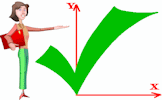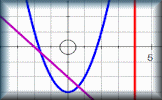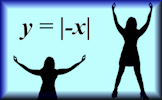# Graph Patterns :: A Coordinated Challenge

##### Level 1 :: Straight line (linear) graphsLevel 2 :: Quadratic graphsMore Graph Activities1

Can you figure out what equations we used to produce the pattern above? Type your answers below.

You don't have to wait until you have entered all of the equations before you click the Draw Graph button.
Sorry, your browser does not support this application. The followined:

This graph plotting challenge is intended to familiarise students with the equation of a straight line. There are five challenges each with between three and six straight lines in a pattern. In order to proceed to the next challenge the correct equation should be entered into the boxes provided.

The graph of any quadratic is a parabola and the equation can be written in the form:

## y = ax2 + bx + c

It is normal to write the terms in descending powers of x. You are encouraged to use the Graph Plotter to investigate how the values of a, b and c affect the shape and position of the graph.

It is probably more convenient to consider the equations in this challenge in the 'completing the square' format:

## y = (x + d)2 + e

The Graph Plotter can also be used to investigate how the values of d and e affect the shape and position of this graph.For example the graph shown to the left is the basic parabola with equation:

## y = x2

Shifting this graph 3 units to the right gives:

## y = (x - 3)2

Shifting the basic parabola 5 units up gives:

## y = x2 + 5

Stretching the basic parabola 2 units in the positive y direction gives:

## y = 2x2

### Related activities

#### Straight Line Graphs10 straight line graph challenges for use with computer graph plotting software or a graphical display calculator.

Transum.org/go/?to=straight

#### Graph MatchMatch the equations with the images of the corresponding graphs. Linear, quadratic and more.

Transum.org/go/?to=graphmatch

#### Human GraphsStudents should be encouraged to stand up and make the shapes of the graphs with their arms.

Transum.org/go/?to=humangraphs

#### Plotting GraphsComplete a table of values then plot the corresponding points to create a linear, quadratic or reciprocal graph.

Transum.org/go/?to=plotting

The solutions to this and other Transum puzzles, exercises and activities are available in this space when you are signed in to your Transum subscription account. If you do not yet have an account and you are a teacher or parent you can apply for one here.